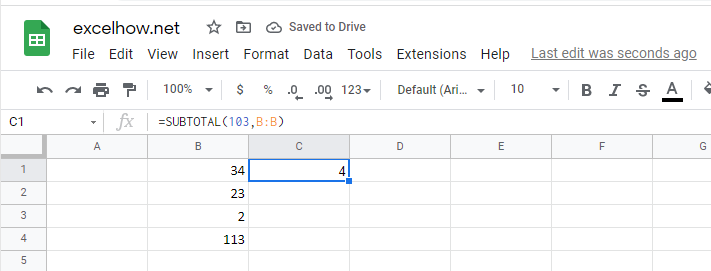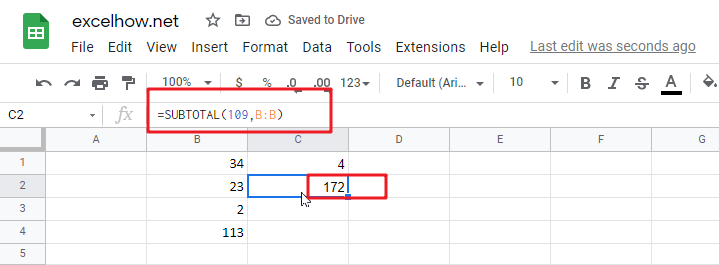# ExcelHow

This post will guide you how to use Google Sheets SUBTOTAL function with syntax and examples in Google Spreadsheet.

## Description

The Google Sheets SUBTOTAL function returns the subtotal of the numbers in a list or database.

The SUBTOTAL function is a build-in function in Google Sheets and it is categorized as a Math and Trigonometry Function.

## Syntax

The syntax of the SUBTOTAL function is as below:

`= SUBTOTAL(function_num, ref1, [ref2])`

Where the SUBTOTAL function argument is:

• Function_num – This is a required argument.  It can be set as 1-11 or 101-111 for the subtotal.  1-11 that includes hidden rows and 101-111 excludes hidden rows.
 Function_num (includes hidden values) Function_num (ignores hidden values) Function 1 101 AVERAGE 2 102 COUNT 3 103 COUNTA 4 104 MAX 5 105 MIN 6 106 PRODUCT 7 107 STDEV 8 108 STDEVP 9 109 SUM 10 110 VAR 11 111 VARP

Ref1– This is a required argument. The first named range or reference that you want to subtotal.

## Google Sheets SUBTOTAL Function Example

the below examples will show you how to use Google Sheets SUBTOTAL function to return the subtotal of the numbers in a list.

#1 =SUBTOTAL(103,B:B)Note: the above formula will call COUNTA function to count the number of cells(B:B) that contain numbers. It will return value 5.

#2 =SUBTOTAL(109,B:B)Note: the above Google Sheets formula will call SUM function to add all numbers in range cell B:B, so it will return value: 160.